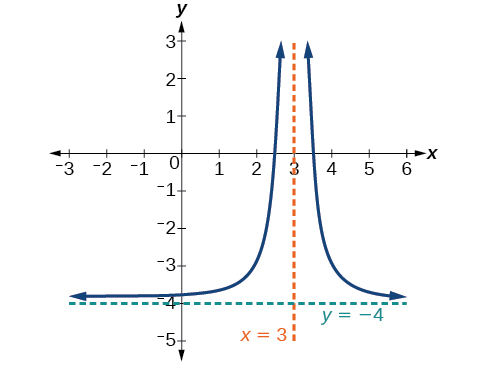# 3.7 Rational functions  (Page 2/16)

 Page 2 / 16

## Vertical asymptote

A vertical asymptote    of a graph is a vertical line $\text{\hspace{0.17em}}x=a\text{\hspace{0.17em}}$ where the graph tends toward positive or negative infinity as the inputs approach $\text{\hspace{0.17em}}a.\text{\hspace{0.17em}}$ We write

## End behavior of $\text{\hspace{0.17em}}f\left(x\right)=\frac{1}{x}$

As the values of $\text{\hspace{0.17em}}x\text{\hspace{0.17em}}$ approach infinity, the function values approach 0. As the values of $\text{\hspace{0.17em}}x\text{\hspace{0.17em}}$ approach negative infinity, the function values approach 0. See [link] . Symbolically, using arrow notation

Based on this overall behavior and the graph, we can see that the function approaches 0 but never actually reaches 0; it seems to level off as the inputs become large. This behavior creates a horizontal asymptote , a horizontal line that the graph approaches as the input increases or decreases without bound. In this case, the graph is approaching the horizontal line $\text{\hspace{0.17em}}y=0.\text{\hspace{0.17em}}$ See [link] .

## Horizontal asymptote

A horizontal asymptote    of a graph is a horizontal line $\text{\hspace{0.17em}}y=b\text{\hspace{0.17em}}$ where the graph approaches the line as the inputs increase or decrease without bound. We write

## Using arrow notation

Use arrow notation to describe the end behavior and local behavior of the function graphed in [link] .

Notice that the graph is showing a vertical asymptote at $\text{\hspace{0.17em}}x=2,\text{\hspace{0.17em}}$ which tells us that the function is undefined at $\text{\hspace{0.17em}}x=2.$

And as the inputs decrease without bound, the graph appears to be leveling off at output values of 4, indicating a horizontal asymptote at $\text{\hspace{0.17em}}y=4.\text{\hspace{0.17em}}$ As the inputs increase without bound, the graph levels off at 4.

Use arrow notation to describe the end behavior and local behavior for the reciprocal squared function.

End behavior: as Local behavior: as (there are no x - or y -intercepts)

## Using transformations to graph a rational function

Sketch a graph of the reciprocal function shifted two units to the left and up three units. Identify the horizontal and vertical asymptotes of the graph, if any.

Shifting the graph left 2 and up 3 would result in the function

$f\left(x\right)=\frac{1}{x+2}+3$

or equivalently, by giving the terms a common denominator,

$f\left(x\right)=\frac{3x+7}{x+2}$

The graph of the shifted function is displayed in [link] .

Notice that this function is undefined at $\text{\hspace{0.17em}}x=-2,\text{\hspace{0.17em}}$ and the graph also is showing a vertical asymptote at $\text{\hspace{0.17em}}x=-2.$

As the inputs increase and decrease without bound, the graph appears to be leveling off at output values of 3, indicating a horizontal asymptote at $\text{\hspace{0.17em}}y=3.$

Sketch the graph, and find the horizontal and vertical asymptotes of the reciprocal squared function that has been shifted right 3 units and down 4 units.The function and the asymptotes are shifted 3 units right and 4 units down. As $\text{\hspace{0.17em}}x\to 3,f\left(x\right)\to \infty ,\text{\hspace{0.17em}}$ and as $\text{\hspace{0.17em}}x\to ±\infty ,f\left(x\right)\to -4.$

The function is $\text{\hspace{0.17em}}f\left(x\right)=\frac{1}{{\left(x-3\right)}^{2}}-4.$

## Solving applied problems involving rational functions

In [link] , we shifted a toolkit function in a way that resulted in the function $\text{\hspace{0.17em}}f\left(x\right)=\frac{3x+7}{x+2}.\text{\hspace{0.17em}}$ This is an example of a rational function. A rational function is a function that can be written as the quotient of two polynomial functions. Many real-world problems require us to find the ratio of two polynomial functions. Problems involving rates and concentrations often involve rational functions.

difference between calculus and pre calculus?
give me an example of a problem so that I can practice answering
x³+y³+z³=42
Robert
dont forget the cube in each variable ;)
Robert
of she solves that, well ... then she has a lot of computational force under her command ....
Walter
what is a function?
I want to learn about the law of exponent
explain this
what is functions?
A mathematical relation such that every input has only one out.
Spiro
yes..it is a relationo of orders pairs of sets one or more input that leads to a exactly one output.
Mubita
Is a rule that assigns to each element X in a set A exactly one element, called F(x), in a set B.
RichieRich
If the plane intersects the cone (either above or below) horizontally, what figure will be created?
can you not take the square root of a negative number
No because a negative times a negative is a positive. No matter what you do you can never multiply the same number by itself and end with a negative
lurverkitten
Actually you can. you get what's called an Imaginary number denoted by i which is represented on the complex plane. The reply above would be correct if we were still confined to the "real" number line.
Liam
Suppose P= {-3,1,3} Q={-3,-2-1} and R= {-2,2,3}.what is the intersection
can I get some pretty basic questions
In what way does set notation relate to function notation
Ama
is precalculus needed to take caculus
It depends on what you already know. Just test yourself with some precalculus questions. If you find them easy, you're good to go.
Spiro
the solution doesn't seem right for this problem
what is the domain of f(x)=x-4/x^2-2x-15 then
x is different from -5&3
Seid
All real x except 5 and - 3
Spiro
***youtu.be/ESxOXfh2Poc
Loree
how to prroved cos⁴x-sin⁴x= cos²x-sin²x are equal
Don't think that you can.
Elliott
By using some imaginary no.
Tanmay
how do you provided cos⁴x-sin⁴x = cos²x-sin²x are equal
What are the question marks for?
ElliottByByBy David CoreyBy IES PortalBy Stephen VoronBy Saylor FoundationBy Jessica CollettBy OpenStaxBy Brooke DelaneyBy Madison ChristianBy Tod McGrathBy OpenStax